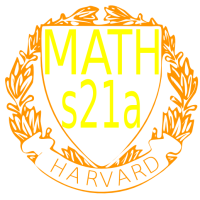Course 30189: Maths21A Summer 2010
Multivariable Calculus
FAQ
Office: SciCtr 434
Send questions of potential general interest to math21b@fas.harvard.edu.

 Question:Do we have to worry about dividing by zero in the Lagrange method if we get rid of the lambda parameter by dividing the first equation by the second Answer: When dividing out lambda by dividing the first equation by the second, you actually do nothing dangerous if you afterwards multiply out again. In two dimensions, you will get from ``` f_x = lambda g_x f_y = lambda g_y ``` that f_x/f_y = g_x/g_y which means f_x g_y - f_y g_x = 0 This just means that the cross product between the two vectors is zero that they are parallel. The Lagrange equations could also have been written as ``` nabla f x nabla g = 0 g = c ``` which is in some sense better since the case nabla g=0 is covered by this. The reason that this is not replacing the Lagrange equations is that the Lagrange equations hold in any dimensions and with arbitrarily many constraints. It is a powerful general tool even so in the cases we usually look at to practice, other methods would work too. Question:Is it ok to use an older edition of a textbook? Answer: It is not really necessary to use a text book. Look around and take, what suits you. We will not follow a textbook nor use homework from textbooks so that it is fine to use an older edition. Newer editions tend to be more reliable and are smoothed out. Books like Stewart also resell well again.